# IntelliJ IDEA签名如何更改

• 更改方法名称。
• 更改方法返回类型。
• 添加新参数并删除现有参数。
• 将默认值分配给参数。
• 重新排序参数。
• 更改参数名称和类型。
• 通过方法调用层次结构传播新参数。

• Java、PHP、JavaScript 和 ActionScript 支持更改方法签名重构。
• 您可以从 UML 类图访问此重构。
• 该更改方法签名重构适用于构造函数。但是在这种情况下，不能更改名称和返回类型。

## 示例

``````// 函数 paint() 在 IShape 接口中声明
public interface IShape {
function paint(g: Graphics): void;
}
// 然后在 Canvas 类的 paint() 函数中调用这个函数
public class Canvas {
private var shapes: Vector.<IShape>;
public function paint(g: Graphics): void {
for each (var shape: IShape in shapes) {
shape.paint(g);
}
}
}
// 现在, 我们将显示 IShape 接口的函数 paint () 的更改签名重构的示例``````

``````// 在此重构示例中, 我们更改了现有参数的名称, 并引入了两个新参数。请注意, 第一个新参数是必需的参数, 而第二项是可选的, 因为它的默认值是在函数定义中指定的。

public interface IShape {
function paint(graphics:Graphics, wireframe:Boolean, offset:Point = null):void;
}

// 执行此重构时, 新参数被传播到 Canvas 类的 paint() 函数中。因此, Canvas.paint()的签名已更改。还要注意如何在 Canvas.paint() 中 IShape.paint() 是怎么调用的。``````
public class Canvas {
private var shapes: Vector.<IShape>;

public function paint(g:Graphics, wireframe:Boolean): void {
for each (var shape: IShape in shapes) {
shape.paint(g, wireframe);
}
}
}
// 此重构的其他结果是可能的。有关详细信息, 请参阅下面的讨论。``````

``````# 此函数将被重命名:
def fibonacci( n ):
a, b = 0, 1
while b < n:
print( b )
a, b = b, a+b

n = int(input("n = "))
fibonacci( n )``````
``````# 使用新名称的函数:
def fibonacci_numbers( n ):
a, b = 0, 1
while b < n:
print( b )
a, b = b, a+b

n = int(input("n = "))
fibonacci_numbers( n )``````

``````# 将添加新参数:
def fibonacci( n ):
a, b = 0, 1
while b < n:
print( b )
a, b = b, a+b

n = int(input("n = ")
fibonacci( n )``````
``````# 函数的新参数，不要忘记指定参数的默认值, 它将用于函数调用。
def fibonacci( n,a,b ):
a, b = 0, 1 # this should be done manually!
while b < n:
print( b )
a, b = b, a+b

n = int(input("n = ")
fibonacci( n,0,1 )``````

• 用于初始化参数的值（或表达式）（IntelliJ IDEA 中的初始值设定项字段）。
• 默认值（或表达式）（ 默认值字段）。

• 如果不使用传播，初始化器值不影响函数调用和调用函数的签名。
• 如果使用传播，则将初始化器值添加到调用函数的定义中作为相应参数的默认值（与您正在更改其签名的函数的方法相同）。

## 更多重构实例

``````public interface IShape {
function paint(g:Graphics, wireframe:Boolean):void;
}
// Canvas 类中的 paint() 函数：
public function paint(g:Graphics, wireframe:Boolean): void
{
for each
(
var shape: IShape in shapes) {
shape.paint(g,wireframe);
}
}``````

``````public interface IShape {
function paint(g:Graphics, wireframe:Boolean):void;
}
// Canvas 类中的 paint() 函数：
public function paint(g:Graphics): void
{
for each
(
var shape: IShape in shapes) {
shape.paint(g,false);
}
}``````

``````public interface IShape {
function paint(g:Graphics, wireframe:Boolean = true):void;
}
// Canvas 类中的 paint() 函数:
public function paint(g:Graphics):
void
{
for each
(
var shape: IShape in shapes) {
shape.paint(g);
}
}``````

``````public interface IShape {
function paint(g:Graphics, wireframe:Boolean = true):void;
}
// Canvas 类中的 paint() 函数:
public function paint(g:Graphics):void
{
for each
(
var shape: IShape in shapes) {
shape.paint(g);
}
}``````

``````public interface IShape {
function paint(g:Graphics, wireframe:Boolean = true):void;
}
// Canvas 类中的 paint() 函数:
public function paint(g:Graphics, wireframe:Boolean = true):
void {
for each
(
var shape: IShape in shapes) {
shape.paint(g,wireframe);
}
}``````

``````public interface IShape {
function paint(g:Graphics,
wireframe:Boolean = true):void;
}
// Canvas 类中的 paint() 函数:
public function paint(g:Graphics):
void {
for each
(
var shape: IShape in shapes) {
shape.paint(g,false);
}
}``````

## 更改方法签名

1. 在编辑器中，将光标放在要更改其签名的方法的名称中。
2. 执行以下操作之一：
• 按 Ctrl+F6。
• 在主菜单上选择：重构|更改签名
• 在上下文菜单上选择：重构| 更改签名。
3. 在 "更改签名" 对话框中，对方法签名进行必要的更改，并指定需要哪些其他相关的更改。您可以：
• 更改方法名称。要做到这一点，请在 "名称" 字段中编辑文本。
• 通过编辑 "返回类型" 字段的内容来更改方法返回类型。只有在 PHP 语言7.1 和更高版本中才能设置方法返回类型。您可以在 php 页面上指定 php 语言级别（文件| 设置| 语言与框架| PHP （Windows 和 Linux 系统）或 IntelliJ IDEA | 首选项| 语言与框架| PHP（macOS系统））。
• 使用表和 "参数" 区域中的按钮管理方法参数：
• 要添加新参数，请单击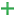并指定相应表行中新参数的属性。添加参数时，可能需要将这些参数传播到调用当前方法的方法。在 PHP 上下文中，当从类的构造函数调用更改签名重构时，新参数可以初始化为一个类字段。要做到这一点，请使用 "创建和初始化类属性" 复选框：
• 选中此复选框后，新添加的参数将作为字段初始化。IntelliJ IDEA 创建与该参数具有相同名称的受保护字段，并添加具有以下赋值的行：
``\$this-><parameter_name> = {#content}lt;parameter_name>;``
• 清除该复选框后，将添加一个没有初始化的参数。
例如，您具有以下构造函数：
``````class ChangeSignatureNewParam {
function __construct() {
\$a = "Constructor in ChangeSignatureNewParam";
print \$a;
}
}``````
如果从 __construct () 方法调用更改签名重构并添加新的 \$q 参数, 则结果将取决于是选中还是清除 "创建和初始化类属性" 复选框：
• 选中 "创建和初始化类属性" 复选框：
``````class ChangeSignatureNewParam {
private \$q;
function __construct(\$q) {
\$a = "Constructor in ChangeSignatureNewParam";
print \$a;
\$this->q = \$q;
}
}``````
• 清除 "创建和初始化类属性" 复选框：
``````class ChangeSignatureNewParam {
function __construct(\$q) {
\$a = "Constructor in ChangeSignatureNewParam";
print \$a;
}
}``````
• 要删除参数，请单击相应行中的任何单元格，然后单击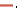• 要重新排序参数，请使用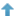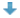按钮。例如，如果要在列表中首先放置某个参数，请单击与该参数对应的行中的任何单元格，然后单击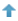所需的次数。
• 要更改参数的名称、类型、初始化程序或参数的默认值，请在参数表中（分别在字段名称类型、初始化器和默认值）进行必要的编辑。
• 沿调用当前方法的方法的层次结构传播新的方法参数（如果有）。
（可能有方法调用您正在更改其签名的方法，这些方法反过来可能会被其他方法调用，等等，您可以通过以下方法将您所做的更改传播到当前方法的参数：调用方法的层次结构，并指定哪些调用方法应该受到影响，哪些不应该。）
传播一个新参数的步骤：
• 单击 "传播参数" 按钮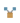• 在左边的窗格中选择“传播新参数的方法” 对话框，展开必要的节点，并选中要将新参数传播到的方法旁边的复选框。为了帮助您选择必要的方法，调用方法的代码和被调用的方法显示在对话框的右侧部分（分别在调用方法和被调用方法窗格中）。当您在左侧窗格中的方法之间切换时，右侧窗格中的代码会相应地更改。
• 单击 "确定"。
4. 要立即执行重构，请单击“重构”。若要在实际执行重构之前查看预期的更改并进行必要的调整，请单击 "预览"。

App下载